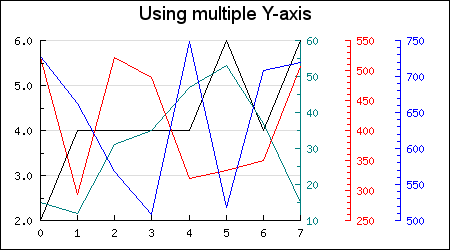## 14.6. Using multiple y-axis

With this feature an arbitrary number of Y-axis can be added to the right side of the graph. The library itself doesn't impose any restrictions on the number of extra Y-axis but from a practical concern it is most likely very difficult to interpret a graph with more than 2-3 extra y-axis.

If there is only need for one more Y axis then the concept of the additional Y2 axis is available as a convenient shortcut for this the most common use of an extra y-axis. The Y2 axis is also a first class citizen in the library meaning it has all the properties available to the "normal" Y-axis.

These extra axis inherits most of the properties of the normal Y-axis (but not all) and the few restrictions imposed are described in Section 14.6.3 below.

Figure 14.17 shows a basic example of how to use this feature. The color encoding maps a specific line to the corresponding axis.

Figure 14.17. Basic example of multiple y-axis `(mulyaxisex1.php)`Adding additional Y-axis is very similar to the way standard Y axis work. The Y-axis are numbered [0..n] where the 0:th axis is the Y-axis furthest to the left. At the same time as these additional Y-axis are used it is also possible to add a Y2 axis. The difference being that the Y2 axis can have all the same options as the Y axis.

For basic usage only three new methods are needed

1. `Graph::SetYScale(\$aNbr,\$aScaleType,\$aMin,\$aMax)`

Specifies the type of scale ('lin', 'int' or 'log') to use for the axis number '`\$aNbr'`

2. `Graph::AddY(\$aNbr,\$aPlot) `

Add a plot to axis number `'\$aNbr' `

3. `Graph::SetYDeltaDist(\$aDistance) `

This is an optional method that if used specifies the default number of pixels between each additional Y-axis. This value will be used unless a specific position for the N:th axis has been specified. By default the additional Y-axis are separated with 50 pixels (which is what is used in Figure 14.17

In order to initialize the extra y-axis the method SetYScale() must be called. In Figure 14.17 the following lines are used

 ```1 2 3 ``` ```\$graph->SetYScale(0,'lin'); \$graph->SetYScale(1,'lin'); \$graph->SetYScale(2,'lin');```

Once setup these additional Y-axis are accessed through the array

`Graph::ynaxis[] `

The axis are numbered from 0. By accessing the axis through this array most of the same method as for the usual Y and Y2 axis are available. For example, the line below will set the color of axis number 1

 ```1 ``` `\$graph->ynaxis->SetColor('red');`

Finally the plots are added to a specific axis with a call to the method `AddY()` (as opposed to the regular `Add()` or `AddY2()` methods). The first argument must be an ordinal that specified the number of the axis that the plot should be added to.

### 14.6.2. Using CSIM together with multiple y-axis

Client Side Image Maps is fully supported and is used in the same way as CSIM for the basic Y-axis. A short example will clarify this. The following code snippet shows a line plot where we have added some oversized markers (in the shape of diamonds) that will act as the image map areas for each data series

 ```1 2 3 4 5 6 7 8 9 ``` ```mark->SetType(MARK_DIAMOND); \$lp2->mark->SetWidth(15); \$lp2->SetCSIMTargets(\$targ2,\$alts2); \$graph->AddY(0,\$lp2); ... ?>```

As usual the targets for the image maps are specified with a call to `Plot::SetCSIMTargets()`. An example of CSIM with multiple y-axis is included in the Example directory and the resulting image is reproduced in Figure 14.18 (but just as an image not as a CSIM image)

Figure 14.18. Illustration of `mulyaxiscsimex1.php`### 14.6.3. Restrictions with multiple y-axis

• Only standard plots can be added to the extra Y-axis, this means no Text objects, no PlotBand, no PlotLines, no Icons etc

• No Grid lines can not be added to the extra Y-axis